# Formatting - Time Hour Minute Seconds Millisecond

Wikitechy | 1698 Views | javascript | 05 Jun 2016

• In javascript the getTime – method is used to return the current time in milliseconds.
• The getHours – method is used to return the current time in hours (0 to 23).
• The getMinutes – method is used to return the minutes (0 to 59).
• The getSeconds – method is used to return the seconds (0 to 59).
• The getMilliseconds – method is used to return the milliseconds (0 to 999).

#### Sample Code :

```<script type="text/javascript">
var date_var = new Date();
var curr_hour = date_var.getHours();
var curr_min = date_var.getMinutes();
var curr_sec = date_var.getSeconds();
var curr_msec = date_var.getMilliseconds();
document.write("Hour - "+curr_hour + ": Minute - " + curr_min + ": Second - "
+ curr_sec + ": Milliseconds - " + curr_msec);
</script>```

#### Code Explanation :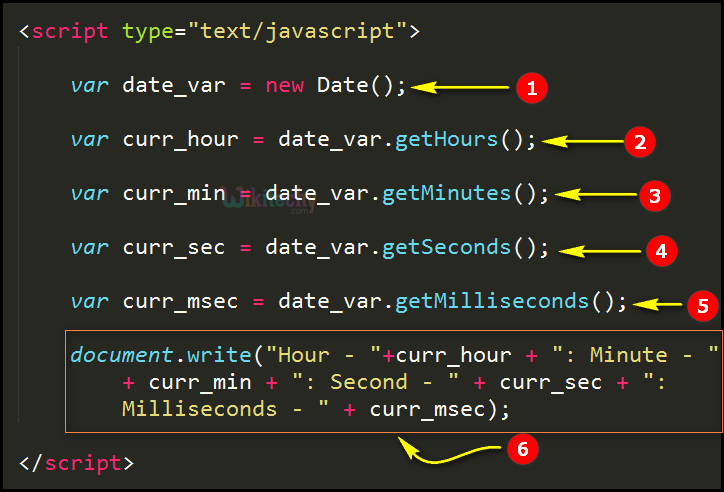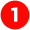Here in this statement we create an object for the date method using new operator and the object name as “date_var”. So, the current date will be stored in the variable “date_var”.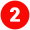In this statement we get the current hour by using the getHours function with the reference of “date_var” object and the current hour’s value will be stored in the variable “curr_hour”.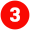In this statement we get the current hour by using the getMinutes function with the reference of “date_var” object and the current minute’s value will be stored in the variable “curr_min”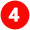In this statement we get the current hour by using the getSeconds function with the reference of “date_var” object and the current minute’s value will be stored in the variable “curr_sec”.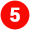In this statement we get the current hour by using the getMilliseconds function with the reference of “date_var” object and the current minute’s value will be stored in the variable “curr_msec”.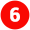Here in this statement “document.write” function is used for write the current hours,minutes,seconds & milliseconds in the browser window by using the concatenation operator” +”.

#### Sample Output :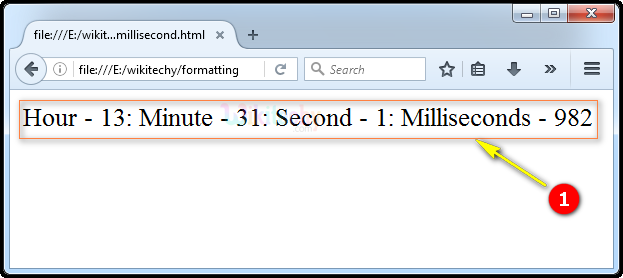Here in this output we have shown the display of the current time as “Hour - 13: Minute - 31: Second - 1: Milliseconds - 982” that is 1-hour, 31-minutes,1-seconds and 982-milliseconds as shown.

Bug Bounty
Webinar